# Car

The car goes from point A to point B at speed 86 km/h and back 53 km/h. If it goes there and back at speed 67 km/h trip would take 10 minutes shorter. What is the distance between points A and B?

Correct result:

s =  258.4 km

#### Solution:We would be pleased if you find an error in the word problem, spelling mistakes, or inaccuracies and send it to us. Thank you!Tips to related online calculators
Looking for calculator of harmonic mean?
Looking for a statistical calculator?
Need help calculate sum, simplify or multiply fractions? Try our fraction calculator.
Do you have a linear equation or system of equations and looking for its solution? Or do you have quadratic equation?
Do you want to convert length units?
Do you want to convert velocity (speed) units?
Do you want to convert time units like minutes to seconds?

## Next similar math problems:

• The machineThe machine was in operation for 9 tenths (in a fraction) of eight hours of working time. How long has he been running?
• Simple equation 6Solve equation with one variable: X/2+X/3+X/4=X+4
• EquationSolve the equation: 1/2-2/8 = 1/10; Write the result as a decimal number.
• CandyPeter had a sachet of candy. He wanted to share with his friends. If he gave them 30 candies, he would have 62 candies. If he gave them 40 candies, he would miss 8 candies. How many friends did Peter have?
• FractionsThree-quarters of an unknown number are 4/5. What is 5/6 of this unknown number?
• Equation with xSolve the following equation: 2x- (8x + 1) - (x + 2) / 5 = 9
• EqnSolve equation with fractions: 2x/3-50=40+x/4
• Simply equationSolve this equation for x: ?
• PlayingHow long have we trained on the pitch when we know that the warm-up took 10 minutes, we trained passes for one-third of the time and we played football half the time?
• SeamstressThe seamstress cut the fabric into 3 parts. The first part was the eighth fabric, the second part was three-fifths of the fabric and the third part had a length of 66 cm. Calculate the original length of the fabric.
• Equation with fractionsSolve equation: ? It is equation with fractions.
• Tourist routeHow long is the tourist route when tourists crossed four-sevenths of the way on foot, crossed the bus twice less than on foot and passed the last 14 kilometers by boat.
• The tourist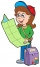The tourist walked a quarter of the way on the first day, a third of the rest on the second day, and 20 km on the last day. How many km did he walk in three days?
• Journey to grandma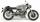Daniel traveled to grandma. He travel 100 km along. Halfway travel by train, one quarter by bus, one tenth by the bicycle and the rest travel by car. How many km travel km by car?
• Poplar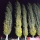How tall is a poplar by the river, if we know that 1/5 of its total height is a trunk, 1/10th of the height is the root and 35m from the trunk to the top of the poplar?
• Steamer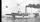At 6 hours 40 minutes steamer sailed from the port at speed 12 km/h. At exactly 10 hours started sail motorboat at speed 42 km/h. When motorboat will catch steamer?
• Call of Duty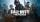Game Call of Duty in CTF (Capture the Flag) opposes the British and the Germans, whose bases are 15 km apart. A German soldier stole the British flag and hid it with it in a secret place between the bases. If the British soldiers set out at a speed of 14